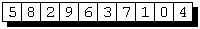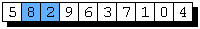## Sunday, February 25, 2007

### Bubble Sort

Note: This is a repost from an old weblog.

Bubble Sort works by iterating through the dataset, comparing two neighboring items at a time, and swapping them if the first item is larger than the second item. In order to sort a dataset of N items, this operation must be done N-1 times. Let me illustrate:

Given:We start by comparing the first two items, 5 and 8:Since 5 is smaller than 8, we don't need to swap them.

Next, we compare the second set of items, 8 and 2:8 is larger than 2, so we have to swap them, resulting in:We keep doing this until we reach the end of the dataset and then we'll start all over again - repeating the process N-1 times (in this example, 9 times since we have 10 items).At the end of our first loop, we end up with:Now we start the whole process over again (we have 8 more loops to go!).Notice anything interesting at the very end? You guessed it! That last comparison is pretty worthless - the last item in the dataset is always going to be the largest value after the first loop, right? Right, but that's not all - each loop through the items, we can ignore more and more items from the end of the dataset (after the second loop, we can ignore the last 2 items; after the third loop, we can ignore the last 3 items and so on). That little trick is our first optimization for this algorithm!

Let's see what the code would look like:

```void
BubbleSort (int a[], int n)
{
int i, j, tmp;

for (i = n - 1; i >= 0; i--) {
for (j = 0; j < i; j++) {
if (a[j] > a[j + 1]) {
tmp = a[j];
a[j] = a[j + 1];
a[j + 1] = tmp;
}
}
}
}
```

Now that we have some code to test, lets see how long it takes to sort a random array of 100,000 integers. To do that, we'll need to write a little program to use our BubbleSort() routine:

```#include <stdlib.h>
#include <time.h>

int main (int argc, char **argv)
{
int array, i;

srand (time (NULL));

for (i = 0; i < 100000; i++)
array[i] = rand ();

BubbleSort (array, 100000);

return 0;
}
```

We'll just use our system's time command to get an estimate of how long it takes to sort.

Go ahead and run our little program a few times. For me, it seems to average about 103 seconds on my AMD Athlon XP 2500.

I don't know about you, but I saw a pretty obvious optimization that we could make to our BubbleSort() implementation that might make it a bit faster. Remember how we noticed that each time through the inner loop, the net result was that the largest item was moved all the way to the right (discounting the largest items from previous loops)? What if, instead of swapping each time through the inner loop, we waited until the inner loop finished and then swapped? Let's try it:

```void
BubbleSort (int a[], int n)
{
int i, j, max, tmp;

for (i = n - 1; i >= 0; i--) {
for (max = 0, j = 1; j < i + 1; j++) {
if (a[j] > a[max])
max = j;
}

if (max < i) {
tmp = a[max];
a[max] = a[i];
a[i] = tmp;
}
}
}
```

In the above code, we use max to hold the index of the largest item we find. We initialize it to the index of the first item (0 in languages like C) and then start our inner for-loop. You'll notice a change here: instead of starting j at 0, we start it at 1 because we've already "looked" at the first item. Also, we need to loop the same number of times - so we continue to iterate as long as j is less than i + 1 (rather than j < i of the previous implementation).

If I now compile our benchmarking program to use this new BubbleSort() implementation, we notice a huge performance increase - or at least I did on my machine. The average execution time for this new implementation seems to be about 51 seconds. That sure beats the pants off 103 seconds, doesn't it?

Even so, 51 seconds to sort 100,000 items is a long wait, especially since they are just simple integers. There's only one more optimization that I can think of. If you think about how bubble sort works, each time through the outer loop, you notice that if we ever go through one of those N-1 iterations without having to swap any items that it is safe to conclude that we're done and so can skip performing the remainder of the N-1 iterations. I'll leave this optimization as an exercise for my readers rather than posting a new implementation here.

Since I don't see any other real obvious optimizations that we can make which would drastically improve performance, I think it's time we consider another sorting algorithm.

To learn more about sorting, I would recommend reading Art of Computer Programming, Volume 3: Sorting and Searching (2nd Edition)#### 1 comment:Anonymous said...

The optimisation listed turns bubble sort in to selection sort - which completely changes its behaviour, include removing the only advantage bubble sort has over some other sorts (linear time for an already, or nearly sorted, lists).